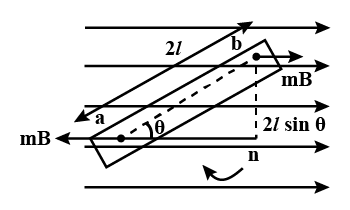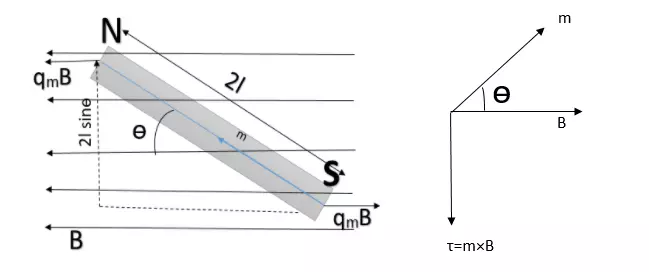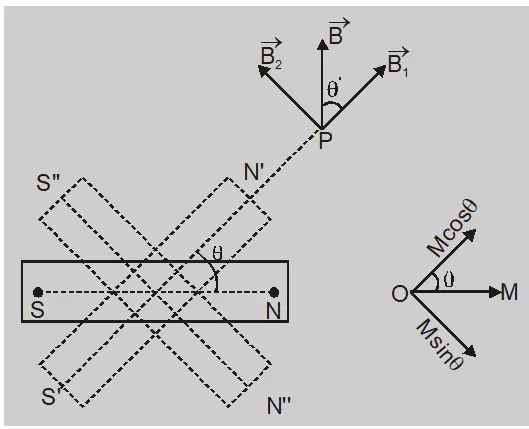# Tag bar magnet

## Vibration magnetometer class 12 | definition, working principle, diagram and usesIn this article, we are going to discuss vibration magnetometer class 12, so let’s get started… What is vibration magnetometer? Definition: A vibration magnetometer is an instrument used to compare the magnetic moment of two bar magnets, and to compare…

## Time period of oscillation of bar magnet in a uniform magnetic field derivationIn this article, we are going to derive an expression for the time period of oscillation of a bar magnet in a uniform magnetic field, so without wasting time, let’s get started… Derivation for the time period of oscillation of…

## Torque on the magnetic dipole (bar magnet) in a uniform magnetic field, class 12In this article, we are going to discuss what if a bar magnet as a magnetic dipole is placed in a uniform magnetic field, so let’s get find out… Torque on the magnetic dipole in an uniform magnetic field [latexpage]Let’s…

## Derive an expression for the magnetic field due to a bar magnet at an arbitrary point.In this article, we are going to derive an expression for the magnetic field due to a bar magnet at an arbitrary point around the bar magnet. So let’s get started… Derivation for the magnetic field due to an bar…